# What’s Required for Your Signal Integrity Application

### By Jon Martens and Bob Buxton

#### Introduction

Signal Integrity applications commonly utilize balanced/differential transmission lines which are typically characterized using vector network analyzers (VNA). There are two approaches to performing these measurements and the selection of the best method depends on what you need to measure.

The “Superposition technique” relies on the inherent linear nature of a transmission line and mathematically derives the differential and common-mode transmission line characteristics through superposition while stimulating just one side of the balanced transmission line at a time. The “True Balanced/Differential technique” uses two sources to create actual differential and common-mode stimuli, hence the shortened name “true balanced”. This white paper offers guidance to signal integrity designers on the differences between these approaches and which one may best fit their need.

#### Measurement Theory

The most complete description for balanced devices are the mixed mode S-parameters (Figure 1). Mixed mode S-parameters are comprised of four blocks of four parameters: differential parameters, common to differential mode parameters, differential to common mode parameters and common mode parameters. These 16 parameters are one way of providing a complete description of a 4-port device. The balanced transmission line does not need to have a ground plane or common terminal. When no ground is physically present, a “virtual” ground is assumed.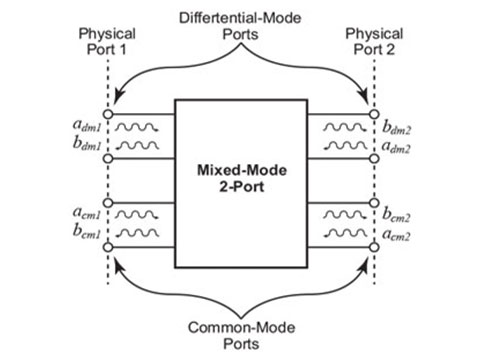(a) Mixed mode two port conceptual diagram.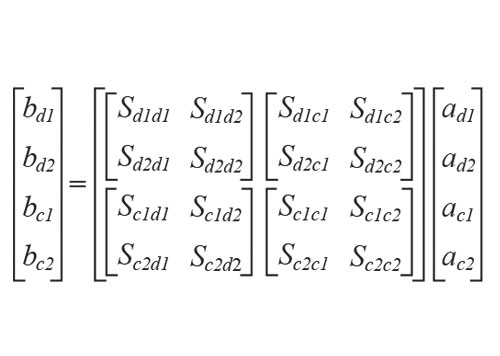(b) Mixed mode s-parameter matrix.

Figure 1. Mixed mode parameters include important mode conversion parameters for determining both the differential and common mode characteristics.

#### Measurement Technique Overview

While both the superposition and true balanced techniques use VNAs, the difference between them lies with the configured options, calibration stability and complexity of the instrument.

For the superposition technique, the mixed mode parameters are mathematically derived from the standard four port S-parameters of the device. This is achieved by measurement of the four port s-parameters as a group of single ended parameters and the use of superposition and relevant impedance transformations to calculate the end result. Superposition requires that the network is linear and time invariant. Due to this principle, the parameters resulting from the first port being stimulated can be combined with the result from the second port (of the differential/common-mode pair) being stimulated to result in the equivalent response from a true balanced or a true common-mode stimulus signal. The execution advantage is that only a single source is required.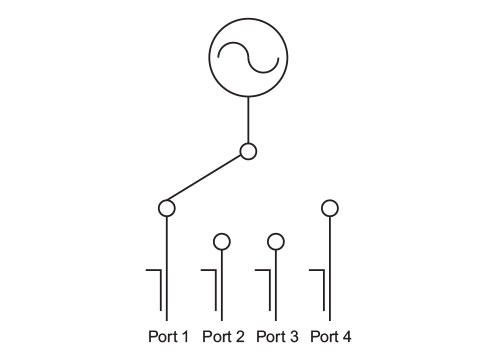Figure 2. The superposition technique uses a standard single source two port VNA with a switch matrix to measure single ended s-parameters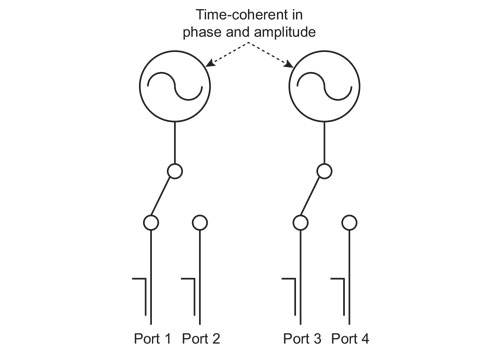Figure 3. The true balanced technique uses a dual source VNA with the sources synchronized with a 180° phase difference to directly measure the common, differential and mixed mode parameters.

The true balanced technique directly measures the differential and common-mode parameters. It requires the use of two sources that are phase coherent and uses active phase and amplitude control of each of the two input sources. An example is shown in Figure 3. In addition, the VNA may have special features such as multipliers, amplifiers and ALC circuitry for precisely controlling each source output. Controlling multiple sources with very precise magnitude and phase alignment also requires a more extensive calibration and can potentially limit the stability of the calibration over time. Further complicating this, the exact phase and amplitude relationship of the signals delivered to the DUT can be a strong function of the DUT reflection characteristics so the phase and amplitudes must be continuously adjusted resulting in further degradation in stability and measurement time. Due to these issues, this technique is usually only employed when required by the device under test.

#### What Do You Need to Measure?

Most signal integrity measurements are of passive components such as transmission lines, PCBs, cables and connectors. For passive devices, the superposition technique is widely accepted to generate the required differential and common-mode responses from a balanced device. Even active devices, if kept in their linear region, can be accurately tested using the superposition technique. For active devices that are driven into compression or saturation, the true balanced technique is required. Table 1 provides a list of components and applicable measurement techniques. Where both techniques are highlighted they will give identical results.

Device to be measured: Superposition Technique True Balanced Technique
Passive Balanced / Differential DUT
Transmission Lines X X
PCB X X
Lumped Components X X
Passive Filters X X
Unshielded and Shielded Twisted Pair, Quad Cables X X
Connectors / Interfaces X X
Linear Active Balanced / Differential DUT
Linear Amplifiers, Differential Amplifiers X X
Linear Active Filters X X
Input / Output Match ADC / DAC X X
Non Linear Active Balanced / Differential DUT
Devices in Compression / Saturation   X
Log Amplifiers   X

Table 1. Superposition offers identical results in most signal integrity applications.

Table 2 highlights the tradeoffs between these two measurement solutions. Most of the tradeoffs are due to the added complexity of the true balanced measurement technique. The true balanced technique requires a VNA with several additional options which add to the overall cost of the test solution. In addition, the modified system calibration also adds a complexity to the measurement process. The phase of the two sources is also susceptible to drift over time and so special and time consuming measurement corrections are made prior to each measurement. Figure 4 highlights the challenges associated with source drift between the two techniques.

Superposition True Balanced
Type of VNA Single source VNA Dual source VNA
Method of obtaining Differential and Mixed Mode Parameters Calculated Measured directly
Type of DUTs Passive and active linear Passive, active linear and non-linear
Available Frequency Range 70 kHz to 110 GHz 10 MHz -67 GHz
Calibration Complexity Typical 4-port Typical 4-port plus calibration
of dual sources
Average Calibration Time T (Time depends on number of points, IF BW, skill of operator) Approx. 2T
Calibration stability considerations Normal measurement calibration intervals Calibrate more frequently due to stability issues
Overall Solution Cost \$ \$\$

Table 2. Comparison of Measurement Techniques.(a) Magnitude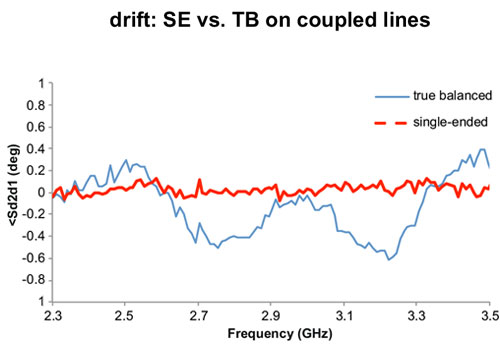(b) Phase

Figure 4. Comparison of the stability of the two techniques highlights the challenges of maintaining alignment between the true differential sources.

#### Conclusion

Determining which measurement technique is best for you is fairly straightforward. If you are measuring passive devices or active devices that stay in their linear range, then the superposition technique offers the simplest, most stable and lowest cost solution. The added cost, calibration complexity and stability concerns of the true balanced solution are only worth it if you are testing active devices in their non-linear range.

Select a country/region